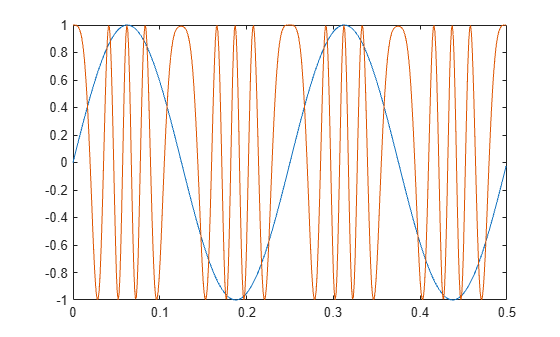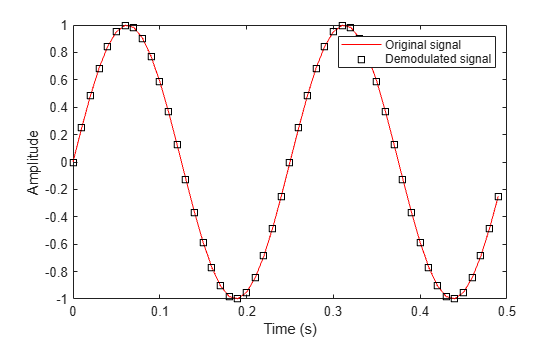Main Content

# comm.FMModulator

Modulate using FM method

## Description

The `FMModulator` System object™ applies FM modulation to an input signal.

To FM modulate a signal:

1. Define and set up the `FMModulator` object. See Construction.

2. Call `step` to apply FM modulation to a signal according to the properties of `comm.FMModulator`. The behavior of `step` is specific to each object in the toolbox.

Note

Starting in R2016b, instead of using the `step` method to perform the operation defined by the System object™, you can call the object with arguments, as if it were a function. For example, ```y = step(obj,x)``` and `y = obj(x)` perform equivalent operations.

## Construction

`H = comm.FMModulator` creates a modulator System object, `H`, that frequency modulates an input signal.

`H = comm.FMModulator(demod)` creates an FM modulator object whose properties are determined by the corresponding FM demodulator object, `demod`.

`H = comm.FMModulator(Name,Value)` creates an FM modulator object with each specified property `Name` set to the specified `Value`. `Name` must appear inside single quotes. You can specify additional name-value pair arguments in any order as (`Name1`,`Value1`,...,`NameN`,`ValueN`).

## Properties

 `FrequencyDeviation` Peak deviation of the output signal frequency (Hz) Specify the frequency deviation of the FM modulator in Hz as a positive real scalar. The default value is `75e3`. The system bandwidth is equal to twice the sum of the frequency deviation and the message bandwidth. This property is nontunable. `SampleRate` Sample rate of the input signal (Hz) Specify the sample rate in Hz as a positive real scalar. The default value is `240e3`. The output sample rate is equal to the input sample rate. This property is nontunable.

## Methods

 step Applies FM baseband modulation
Common to All System Objects
`release`

Allow System object property value changes

`reset`

Reset internal states of System object

When using `reset`, this method resets the windowed suffix from the last symbol in the previously processed frame.

## Examples

collapse all

Apply baseband modulation to a sine wave input signal and plot its response.

Set the example parameters.

```fs = 1e3; % Sample rate (Hz) ts = 1/fs; % Sample period (s) fd = 50; % Frequency deviation (Hz)```

Create a sinusoidal input signal with duration 0.5s and frequency 4 Hz.

```t = (0:ts:0.5-ts)'; x = sin(2*pi*4*t);```

Create an FM modulator System object™.

`MOD = comm.FMModulator('SampleRate',fs,'FrequencyDeviation',fd);`

FM modulate the input signal and plot its real part. You can see that the frequency of the modulated signal changes with the amplitude of the input signal.

```y = step(MOD,x); plot(t,[x real(y)])```Apply FM baseband modulation to a white Gaussian noise source and plot its spectrum.

Set the example parameters.

```fs = 1e3; % Sample rate (Hz) ts = 1/fs; % Sample period (s) fd = 10; % Frequency deviation (Hz) ```

Create a white Gaussian noise source having a duration of 5s.

```t = (0:ts:5-ts)'; x = wgn(length(t),1,0); ```

Create an FM modulator System object? and modulate the input signal.

```MOD1 = comm.FMModulator('SampleRate',fs,'FrequencyDeviation',fd); y = step(MOD1,x); ```

Create another modulator object, `MOD2`, whose frequency deviation is five times larger and apply FM modulation.

```MOD2 = comm.FMModulator('SampleRate',fs,'FrequencyDeviation',5*fd); z = step(MOD2,x); ```

Plot the spectra of the two modulated signals. The larger frequency deviation associated with channel 2 results in a noise level that is 10 dB higher.

```SA = dsp.SpectrumAnalyzer('SampleRate',fs,'ShowLegend',true); step(SA,[y z]) ```Modulate and demodulate a sinusoidal signal. Plot the demodulated signal and compare it to the original signal.

Set the example parameters.

```fs = 100; % Sample rate (Hz) ts = 1/fs; % Sample period (s) fd = 25; % Frequency deviation (Hz)```

Create a sinusoidal input signal with duration 0.5 s and frequency 4 Hz.

```t = (0:ts:0.5-ts)'; x = sin(2*pi*4*t);```

Create FM modulator and demodulator System objects.

```fmmod = comm.FMModulator('SampleRate',fs,'FrequencyDeviation',fd); fmdemod = comm.FMDemodulator('SampleRate',fs,'FrequencyDeviation',fd);```

FM modulate the input signal and plot its real part. You can see that the frequency of the modulated signal changes with the amplitude of the input signal.

```y = fmmod(x); plot(t,[x real(y)])```Demodulate the FM modulated signal.

`z = fmdemod(y);`

Plot the input and demodulated signals. The demodulator output signal exactly aligns with the input signal.

```plot(t,x,'r',t,z,'ks') legend('Input Signal','Demod Signal') xlabel('Time (s)') ylabel('Amplitude')```## Selected Bibliography

 Chakrabarti, I. H., and Hatai, I. “A New High-Performance Digital FM Modulator and Demodulator for Software-Defined Radio and Its FPGA Implementation.” International Journal of Reconfigurable Computing. Vol. 2011, No. 10.1155/2011, 2011, p. 10.

 Taub, Herbert, and Donald L. Schilling. Principles of Communication Systems. New York: McGraw-Hill, 1971, pp. 142–155.

## Algorithms

Represent a frequency modulated passband signal, Y(t), as

`$Y\left(t\right)=A\mathrm{cos}\left(2\pi {f}_{c}t+2\pi {f}_{\Delta }{\int }_{0}^{t}x\left(\tau \right)d\tau \right)\text{\hspace{0.17em}},$`

where A is the carrier amplitude, fc is the carrier frequency, x(τ) is the baseband input signal, and fΔ is the frequency deviation in Hz. The frequency deviation is the maximum shift from fc in one direction, assuming |x(t)| ≤ 1.

A baseband FM signal can be derived from the passband representation by downconverting it by fc such that

`$\begin{array}{c}{y}_{s}\left(t\right)=Y\left(t\right){e}^{-j2\pi {f}_{c}t}=\frac{A}{2}\left[{e}^{j\left(2\pi {f}_{c}t+2\pi {f}_{\Delta }{\int }_{0}^{t}x\left(\tau \right)d\tau \right)}+{e}^{-j\left(2\pi {f}_{c}t+2\pi {f}_{\Delta }{\int }_{0}^{t}x\left(\tau \right)d\tau \right)}\right]{e}^{-j2\pi {f}_{c}t}\\ =\frac{A}{2}\left[{e}^{j2\pi {f}_{\Delta }{\int }_{0}^{t}x\left(\tau \right)d\tau }+{e}^{-j4\pi {f}_{c}t-j2\pi {f}_{\Delta }{\int }_{0}^{t}x\left(\tau \right)d\tau }\right]\text{\hspace{0.17em}}.\end{array}$`

Removing the component at -2fc from ys(t) leaves the baseband signal representation, y(t), which is expressed as

`$y\left(t\right)=\frac{A}{2}{e}^{j2\pi {f}_{\Delta }{\int }_{0}^{t}x\left(\tau \right)d\tau }.$`

The expression for y(t) is rewritten as

`$y\left(t\right)=\frac{A}{2}{e}^{j\varphi \left(t\right)}\text{\hspace{0.17em}},$`

where $\varphi \left(t\right)=2\pi {f}_{\Delta }{\int }_{0}^{t}x\left(\tau \right)d\tau$, which implies that the input signal is a scaled version of the derivative of the phase, ϕ(t).

A baseband delay demodulator is used to recover the input signal from y(t).A delayed and conjugated copy of the received signal is subtracted from the signal itself,

`$w\left(t\right)=\frac{{A}^{2}}{4}{e}^{j\varphi \left(t\right)}{e}^{-j\varphi \left(t-T\right)}=\frac{{A}^{2}}{4}{e}^{j\left[\varphi \left(t\right)-\varphi \left(t-T\right)\right]}\text{\hspace{0.17em}},$`

where T is the sample period. In discrete terms, wn=w(nT), and

`$\begin{array}{l}{w}_{n}=\frac{{A}^{2}}{4}{e}^{j\left[{\varphi }_{n}-{\varphi }_{n-1}\right]}\text{\hspace{0.17em}}\text{,}\\ {v}_{n}={\varphi }_{n}-{\varphi }_{n-1}\text{\hspace{0.17em}}.\end{array}$`

The signal vn is the approximate derivative of ϕn, such that vnxn.

## See Also

Introduced in R2015a

## Support

#### Bridging Wireless Communications Design and Testing with MATLAB

Download white paper